## 5.17Multi-grid method

The methods for solving matrix equations described so far are Gauss-Seidel and CG. They are iterative, and require few iterations to calculate changes in the ﬁeld, when the change at any given point is inﬂuenced only by points in the close vicinity.

This makes them eﬃcient for solving equations whose range of inﬂuence is limited, e.g. by the ﬂow speed through advection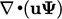in a transport equation.

The pressure equation contains neither a local rate of change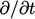nor advection term (assuming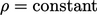). A disturbance at any point inﬂuences the solution everywhere in the domain instantaneously, as discussed in Sec. 4.3 .

To transfer changes across a domain, Gauss-Seidel might require as many sweeps as the number of cells across a domain, which would be impractical. Some method is therefore needed that transfers change across the domain more eﬃciently.

The multi-grid method6 uses a coarse mesh to overcome the problem of slow transfer of change across the domain, reducing the error at “low-frequency”. It transfers that solution through a succession of ﬁner meshes to be accurate at higher frequencies.

The multi-grid method works by ﬁrst calculating the matrix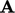and residual vector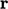on the original (ﬁnest) mesh. Coarser meshes are then successively formed until the mesh reaches its coarsest level, containing as few as 10 cells.

The matrixandare formed at each level of coarsening. Diﬀerent methods exist to calculateand, including a simple agglomeration discussed in Sec. 5.18 .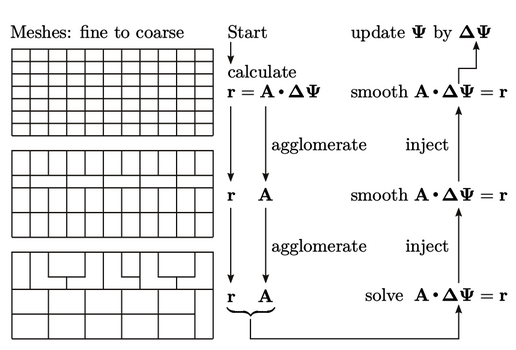Equations are constructed in terms of the correction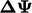, required to make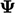exact, related to the residualby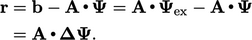(5.36)
At the coarsest level,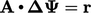is solved precisely for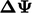, which can be performed eﬃciently using a direct solver since the matrix is small.is then injected into the ﬁner level, providing the initialfor a few sweeps of the Gauss-Seidel method at that level. Smoothing and injection are repeated up to the ﬁnest level, when the ﬁnalis applied to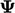to yield the solution.

It is generally more eﬃcient to do more Gauss-Seidel sweeps at the coarser levels, when the cost if low due to a smaller matrix size. For example, 4 sweeps may be applied at the coarser levels, while 2 sweeps are applied at the ﬁnest.

6Achi Brandt, Multi-level adaptive solutions to boundary-value problems, 1977.

Notes on CFD: General Principles - 5.17 Multi-grid method